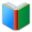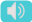# 6th Grade Word List: Math

Words for students of 6th Grade, the page is for math words (List 1), which are essential vocabulary for 6th Grade academic classes.
 AI Writing HelperFree: Paragraph - VIP: Essay
 Actions upon current list

 All lists of current grdae
Spelling Words:

Arts Words:

Social Words:

Math Words:

Science Words:

Literature Words:The SwineherdThe Red ShoesA Study In Scarlet
 base numberthe number that is going to be raised to a power
 circumferenceboundary line of a circle, figure, area, or object
 complementcomplete; consummate; make perfect
 convertchange something into another form; transform
 coordinate planecoordinate system that specifies each point uniquely in a plane by a pair of numerical coordinates
 diameterlength of straight line passing through the center of a circle and connecting two points on the circumference
 evaluatejudge; examine and judge carefully; appraise
 factorizationthe resolution of an entity into factors such that when multiplied together they give the original entity
 non-terminating decimala decimal numeral that does not terminate in an infinite sequence
 numerical expressioncombines numbers, variables, or constants using operators
 pithe ratio of a circle's circumference to its diameter; equal to 3.14159265358979323846...
 plane figurea two-dimensional shape
 prime factorthe prime factors of a quantity are all of the prime quantities that will exactly divide the given quantity
 radiusright line drawn or extending from the center of a circle to the periphery
 reciprocalconcerning each of two or more persons or things; exchangeable; interacting
 sequencesthe action of following in order; serial arrangement in which things follow in logical order or a recurrent pattern
 similaritythe quality of being similar
 simplifymake simple; make less complex; make clear by giving the explanation for
 square unitsunit used to measure area
 substitutionthe act of putting one thing or person in the place of another; an event in which one thing is substituted for another
 supplementadd as something seems insufficient; complement; extension; addition
 terminating decimala number that stops dividing after a number of decimal points and is as a result a rational number
 Quick Access Worksheet:
-->